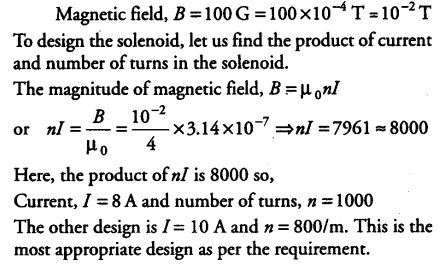# Suggest some appropriate design particulars of a solenoid

A magnetic field of 100 G(1 G = \${{10}^{-4}}\$T) is required which is uniform in a region of linear dimension about 10 cm and area of cross-section about \${{10}^{-3}}\$ \${{m}^{2}}\$. The maximum current carrying capacity of a given coil of wire is 15 A and the number of turns per unit length that can be wound round a core is at most 1000 turns \${{m}^{-1}}\$. Suggest some appropriate design particulars of a solenoid for the required purpose. Assume the core is not ferromagnetic.